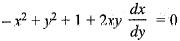Courses

# Differential Equations - 1

## 20 Questions MCQ Test Topic-wise Tests & Solved Examples for IIT JAM Mathematics | Differential Equations - 1

Description
This mock test of Differential Equations - 1 for Mathematics helps you for every Mathematics entrance exam. This contains 20 Multiple Choice Questions for Mathematics Differential Equations - 1 (mcq) to study with solutions a complete question bank. The solved questions answers in this Differential Equations - 1 quiz give you a good mix of easy questions and tough questions. Mathematics students definitely take this Differential Equations - 1 exercise for a better result in the exam. You can find other Differential Equations - 1 extra questions, long questions & short questions for Mathematics on EduRev as well by searching above.
QUESTION: 1

### Let k be real constant. The solution of the differential equations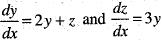satisfies the relation

Solution:

we have dy/dx = 2y + z and ...(i)
dz/dx = 3y  ...(ii)
from 3 (i) l (ii), we get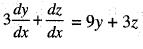implies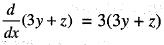on integrating, we get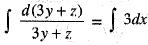implies ln(3y + z) = 3x + c
So, 3y + z = ke3x

QUESTION: 2

### One of the integrating factors of the differential equation (y2 - 3xy)dx + (x2 - xy)dy = 0 is

Solution:

Since, given differential equation is homogeneous
So, I.F =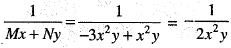QUESTION: 3

### The differential equation 2ydx - (3y - 2x)dy = 0

Solution:

Here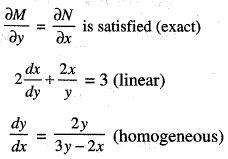QUESTION: 4

The solution of the differential equation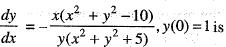Solution:

we have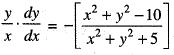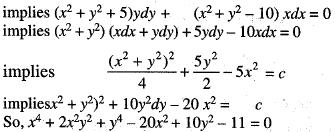QUESTION: 5

Consider the differential equation 2 cos (y2)dx - xy sin (y2)dy = 0

Solution:

we have 2cos (y2)dx - xy sin (y2) dy = 0 Multiplying by x3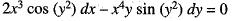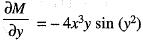and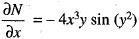Since,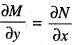So, x3 is integrating factor.

QUESTION: 6

Let f, g : [-1, 1] → R, f (x) = x3, g(x) = x2 IxI Then
y" + py' + qy = 0 on [-1, 1]

Solution:

Here, lxI = -x for x < 0 = x for x ≥ 0
So, g(x) = x3 for x ≥ 0
= -x3 for x < 0
and f(x) = x3

QUESTION: 7

The solution of the differential equation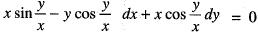C

Solution:

The given differential equation is homogeneous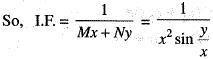Multiplying the differential equations by I.F.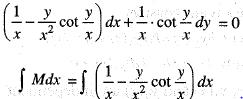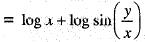...(i)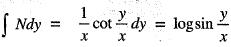...(ii)
Adding (i) and (ii) writing common terms once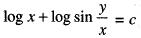implies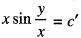QUESTION: 8

An integration factor of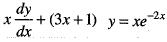is

Solution:

we have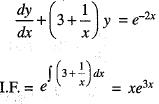QUESTION: 9

The general solution of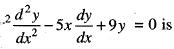(Here c1 and c2 are arbitrary constants.)

Solution:

Let z = log x, Then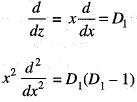The differential equation changes to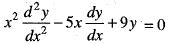implies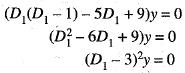So,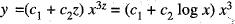QUESTION: 10

If y = (c1 + c2x ) ex is the general solution of the differential equation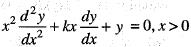then k equals

Solution:

Let log x = z, Then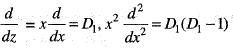The differential equation changes to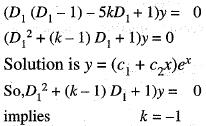QUESTION: 11

Let y1(x) and y2(x) be linearly independent solution of the differential equation y" + P(x) y' + Q(x)y = 0, where P(x) and Q(x) are continuous functions on an interval I. Then y3(x) = ay1(x) + by2(x) and y4(x) = cy1(x) dy2(x) are linearly independent solutions of the given differential equations if

Solution:

y3(x) and y4(x) are linearly dependent if y3(x) = ky4(x) where k is any constant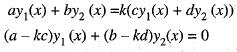Since, y1(x) and y2(x) are independent
So, a - kc = 0
and b - kd = 0
or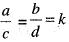So, y3(x) and y4(x) are independent if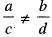QUESTION: 12

Consider the differential equations, satisfying y(0) = 0, where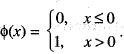This initial value problem

Solution:

we have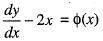or,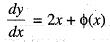Thus,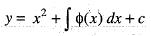implies y = x2 + c for x ≤ 0,
but y(0) = 0 implies c = 0
So,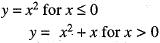QUESTION: 13

The particular integral of the differential equation
y" + y' + 3y = 5 cos(2x + 3) is

Solution:

we have (D2 + D + 3)y = 5 cos(2x + 3)
So,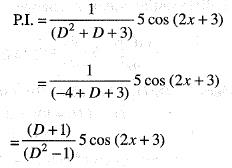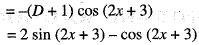QUESTION: 14

The general solution of differential equation
4x2y" - 8xy' + 9y = 0 is

Solution:

Let z = log x, Then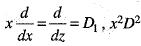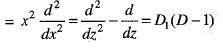So, The differential equation becomes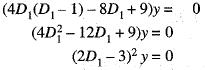So,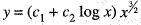QUESTION: 15

Let 1, x and x2 be the solutions of a second order linear non-homogeneous differential equation on -1 < x < 1. Then its general solution, involving arbitrary constants c1 and c2, can be written as

Solution:

QUESTION: 16

If g(x, y)dx + (x + y )dy = 0 is an exact differential equation and if g(x, 0) = x2, then the general solution of the differential equation is

Solution:

g(x, y)dx + (x + y) dy = 0 is exact if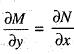implies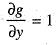g (x , 0) = x2
So, g(x, y) = x2 + y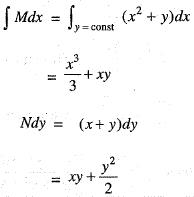So, general solution is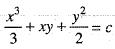implies 2x3 + 6xy + 3y2 = c

QUESTION: 17

A general solution of the differential equation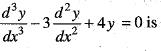Solution: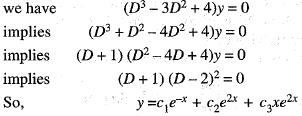QUESTION: 18

Consider the differential equation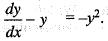Then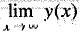is equal to

Solution:

we have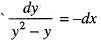implies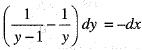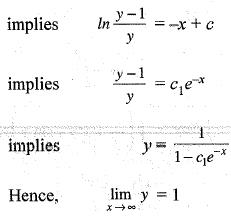QUESTION: 19

Consider the differential equation dy/dx = ay - by2,  where a, b > 0 and y(0) = y0.  As  x → + ∝, the solution y (x) tends to

Solution: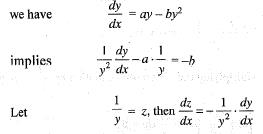So, Equation becomes,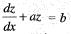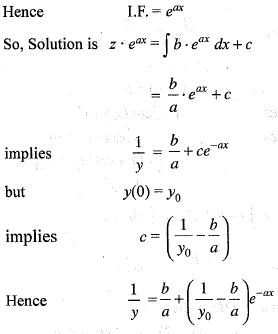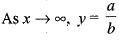QUESTION: 20

Orthogonal trajectories of the family of curves (x - 1)2 + y2 + 2ax = 0 are the solution of the differential equation

Solution:

we have (x - 1)2 + y2 + 2ax = 0 ...(i)
Differentiating w.r.t. x, we get
2(x - 1) + 2y y' + 2a = 0
implies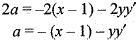Putting expression of a in eq. (i), we get
(x - 1)2 + y2 - 2x((x - 1) + yy') = 0
implies x2 - 2x + 1 + y2 - 2x2 + 2x + 2xyy' = 0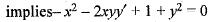Orthogonal trajectories are given by